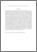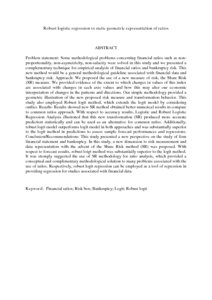# Robust logistic regression to static geometric representation of ratios

## Citation

Bahiraie, Alireza and Ibrahim, Noor Akma and Abdul Karim, Mohd Azhar and Mohd, Ismail (2009) Robust logistic regression to static geometric representation of ratios. Journal of Mathematics and Statistics, 5 (3). pp. 226-233. ISSN 1549-3644; ESSN: 1558-6359

## Abstract

Problem statement: Some methodological problems concerning financial ratios such as non-proportionality, non-asymetricity, non-salacity were solved in this study and we presented a complementary technique for empirical analysis of financial ratios and bankruptcy risk. This new method would be a general methodological guideline associated with financial data and bankruptcy risk. Approach: We proposed the use of a new measure of risk, the Share Risk (SR) measure. We provided evidence of the extent to which changes in values of this index are associated with changes in each axis values and how this may alter our economic interpretation of changes in the patterns and directions. Our simple methodology provided a geometric illustration of the new proposed risk measure and transformation behavior. This study also employed Robust logit method, which extends the logit model by considering outlier. Results: Results showed new SR method obtained better numerical results in compare to common ratios approach. With respect to accuracy results, Logistic and Robust Logistic Regression Analysis illustrated that this new transformation (SR) produced more accurate prediction statistically and can be used as an alternative for common ratios. Additionally, robust logit model outperforms logit model in both approaches and was substantially superior to the logit method in predictions to assess sample forecast performances and regressions. Conclusion/Recommendations: This study presented a new perspective on the study of firm financial statement and bankruptcy. In this study, a new dimension to risk measurement and data representation with the advent of the Share Risk method (SR) was proposed. With respect to forecast results, robust loigt method was substantially superior to the logit method. It was strongly suggested the use of SR methodology for ratio analysis, which provided a conceptual and complimentary methodological solution to many problems associated with the use of ratios. Respectively, robust logit regression can be employed as a tool of regression in providing regression for studies associated with financial data.Preview
PDF (Abstract)
Robust logistic regression to static geometric representation of ratios.pdfView Item